Learn to Code via Tutorials on Repl.it!

← Back to all posts
Fibonacci! 🌀

# G'day People!

Fourth week of school, done!
Now I can relax in a weekend drowned in homeowork! (yay?)

Anywaysssss...

# Welcome to a tutorial on The Fibonacci Sequence!

I will be covering who Leonardo Pisano Bigollo is, what one of his most famous work is about, and I've also included a quick repl where you can get as much terms (numbers) of the sequence you want! There will also be some interesting facts at the end.

All right, let's start!

## Leonardo Pisano Bigollo

Leonardo Pisano Bigollo was an Italian mathematician who lived from 1170 to 1250 (a longggg time ago!). He is best known thought as Fibonacci.

His works include of course the Fibonacci Sequence, but he also introduced to the world math concepts like the Arabic numbering system, the concept of square roots, number sequencing, and also math word problems.

## The Fibonacci Sequence

The Fibonacci Sequence, named after the mathematician who discovered it, is a series of numbers. The next number is found by adding the 2 numbers before it.

The first numbers are:

`0, 1, 1, 2, 3, 5, 8, 13, 21, 34, 55, etc.`

As you can see, the 3rd number in the sequence is `1`, made with adding `0` and `1`, the 2 numbers behind it. Then `2` is found by adding `1` and `1`. [...] `55` is found by adding `21` and `34`, and so on and so forth.

#### Golden Spiral

Now if we take the widths (the numbers) and make squares out of them, and then if we make arcs going from one corner to the other corner, we get a lovely spiral:As you can see, the first 2 squares in brown and purple have a width of 1, then the square in pink has a width of 2, next the square in dark blue has a width of 3, [...], and the square in red has width of of 34. Of course the loop goes on until infinty, so I havn't represented everything, but it's just to show the beginning.

###### (and sorry it's not that precise, here is a better one from google:)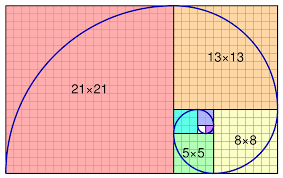The important part of this spiral is that we can find it several times in nature:Go here to find more out about the Golden Ratio!

#### Formula

First, we have to number the series from 0:

So for example term number 0 is 0, term 1 is 1, term 7 is 13, and so on.

This means that term 6 (8) = term 4 (3) + term 5 (5). We can represent it with a table with the first numbers of the Fibonacci: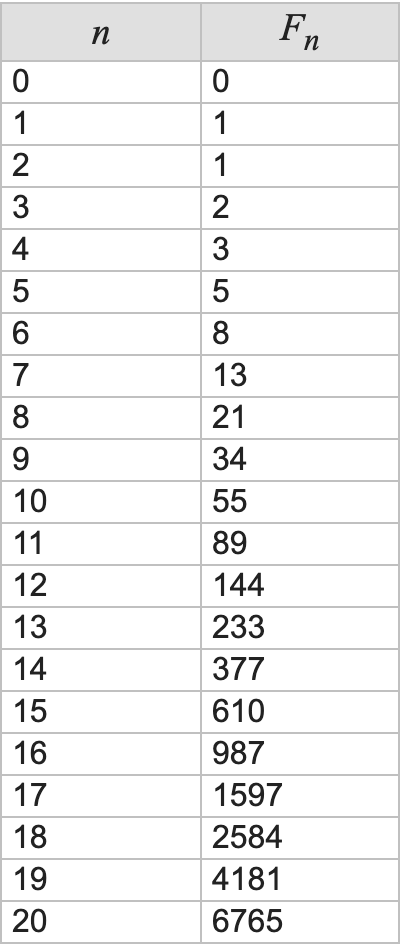So now we can write the formula: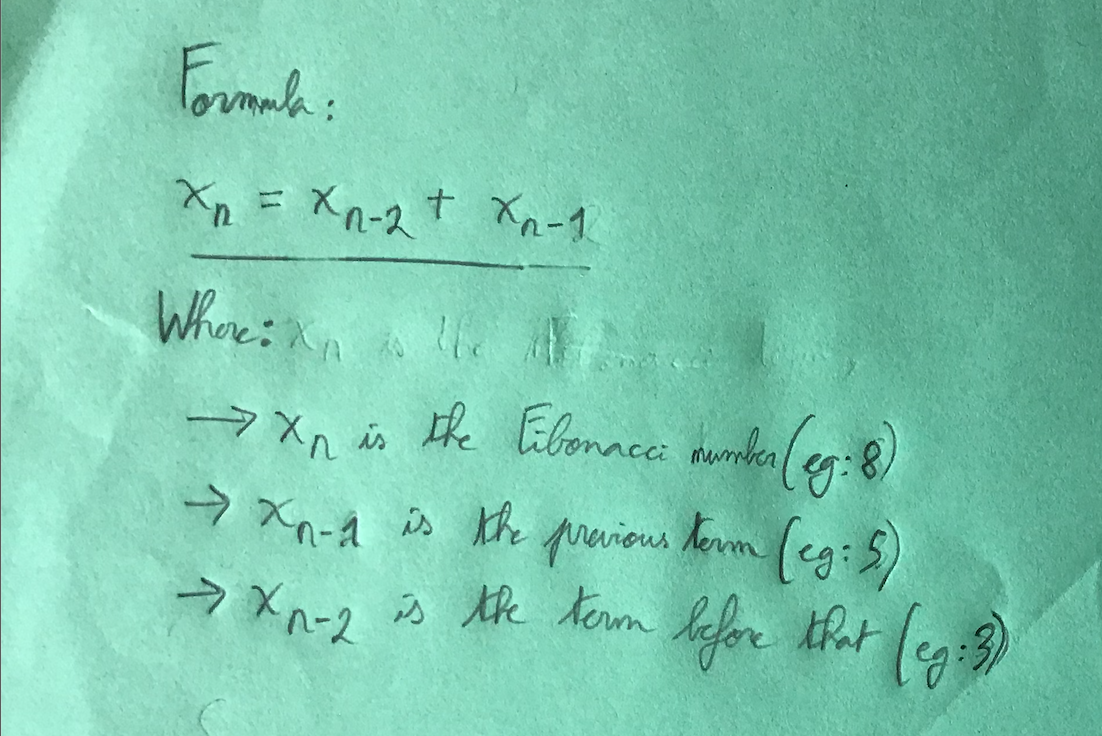Now with an example, let's calculate term 10 (which is 55):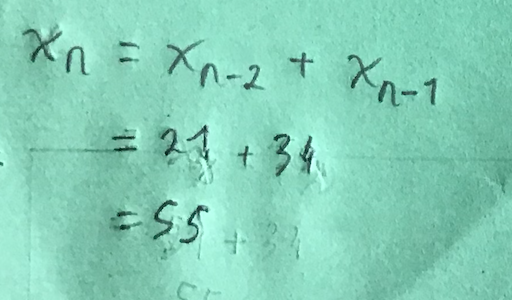## Interesting facts

#### Multiples

Every 3rd number of the Fibonacci Series is a multiple of 2: 2, 8, 34, etc.

Every 4th number of the Fibonacci Series is a multiple of 3: 3, 21, 144, etc.

Every 5th number of the Fibonacci Series is a multiple of 5: 5, 55, 610, etc.

And so on and so forth.
We can sum this up with the following: every nth is a multiple of Xn.

To visualize this better: back to the table with the numbers:As you can see, the 3rd number of the Fibonacci (starting at 1) is 2, and every 2nd number starting at 0 (2, 8, 34, etc) is a multiple of 2.

Another example:

The 5th number of the Fibonacci is 5, and every 5th number is a multiple of 5 (5, 55, 610, 6765, etc.)

#### Fibonacci Day

Fibonacci day is November 23rd; if you write like this: 11/23, we get the first 4 digits of the sequence.

#### Miles and Kilometers.

Taking the first few terms of the sequence:

`0, 1, 1, 2, 3, 4, 8, 13, 21, 34, 55, etc.`

If we take 2 consecutive numbers, we get the 1st number in miles, and the second number the equivalent in kilometers (roughly):

``````0 mi = 0 km
1 mi = 1.61 km ≈ 1
1 mi = 1.61 km ≈ 2
2 mi = 3.22 km ≈ 3
3 mi = 4.83 km ≈ 4
[...]
21 mi = 33.8 km ≈ 34
34 mi = 54.72 km ≈ 55
55 mi = 88.51 km ≈ 89
etc. ``````

As you can see, each number is the sum of the 2 numbers before it. The higher we go, the more precise it is.

#### Honeybee

This one's a bit more complex, but neverless still interesting:

A more real life, found in nature example is the ancestral lineage of the Honeybee.

In a Honeybee colony there are three types of bees:

Queen Bee — A single Female that produces eggs
Worker Bees — Females that do work and produce no eggs
Drone Bees — Males that do no work

♀ Female Honeybees are produced from the Queen’s fertilized eggs, so they have two parents, one Female and one Male

♂ Male Drone Honeybees are produced from the Queen’s unfertilized eggs, so they only have one parent, a Female

When you trace back the ancestral tree of any Honeybee (Male or Female), you’ll see that the number of bees in each prior generation matches the numbers in the Fibonacci sequence.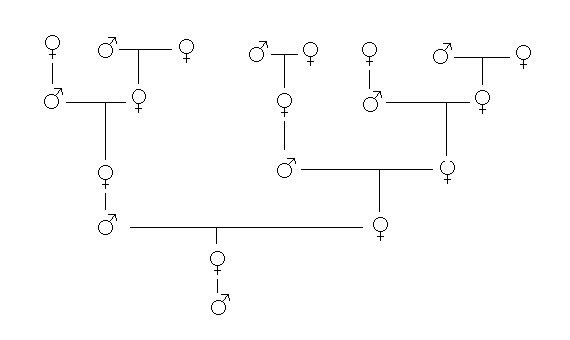(from here)

#### 1/89

`0.01123595506...`

Notice how the first 6 digits (1, 1, 2, 3, 4, 5).

However, if you write the fraction a different way:

``````0.0
0.01
0.001
0.0002
0.00003
0.000005
0.0000008
0.000000013
0.0000000021
0.00000000033
etc.``````

You see that the numbers at the end are the terms of the Fibonacci. However, they're overlapping; if you add those numbers above, you'll get an approximation of `1/89` (something like 0.011235955056179775.), and the more deeper you go, the more precise result you'll get, and therefore the more Fibonacci terms you'll get.

### The Program

#### Output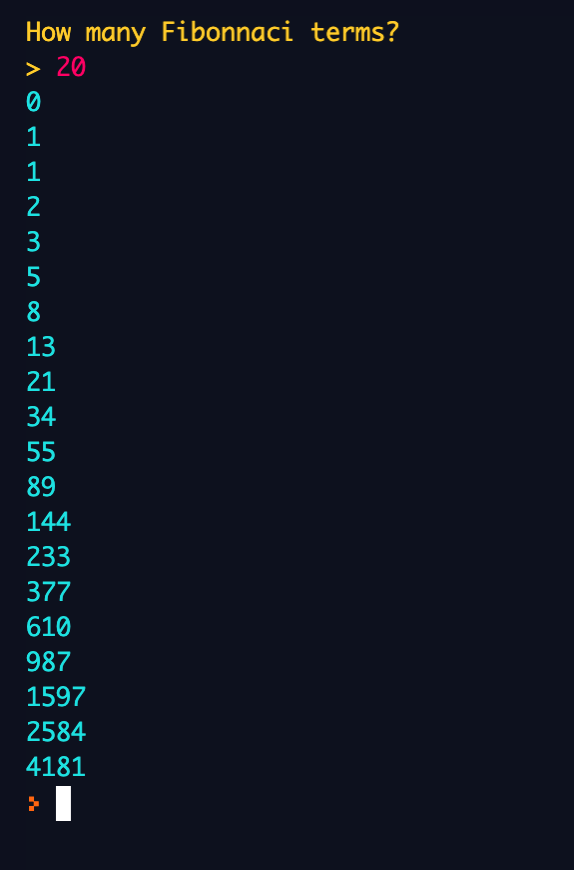So the program will ask you how many Fibonacci terms you want. Remember that if you enter a value too big, it'll take a pretty long time and it might crash lol.

#### Code

The code is pretty straightforward, I also added comments so you can understand it better. I have the basic code, but also added some colors, and also a `try` and `except` in case the user types something else than integers, and also a `while True` loop so that it loops forever.

First there's two variables `x1` and `x2` that have the initial value of `0` and `1`, respectively. Then there's an `input` to ask the user how many terms they want. Next there's a `for` loop that repeats for the number of times the user said before.

Afterwards, there's a `print` statement that outputs the Fibonacci term (with color). Then, it makes a new variable called `next_x` which is the sum of the previous terms, `x1` and `x2`, like in the Fibonacci sequence. And then, `x1` becomes `x2`, and `x2` becomes `next_x`. This is to kind of "advance" the numbers; put all the numbers forward.

Here my sources:

All right, well, guess that's all lol! Hope you've learnt something and enjoyed the beautiful colors of the repl!

Now you can impress all your friends and family with these amazing facts!

🌀 🌀 🌀 🌀

# Everyone have a super-duper day!

Cya next time! :D

SixBeeps (3064)

Given that you gave the recursive formula for the sequence, I think it might've been better to implement a recursive function for it as well.

``````def fib(n):
if (n <= 1):
return 1
return fib(n - 1) + fib(n - 2)``````
Bookie0 (4007)

ah yes lol I could have done that! The reason its like this is because I had made the program with all the comments first, then did the tutorial and discovered the formula after lol

but thanks anyways! :) @SixBeeps

LTI2 (95)

i made one pretty much like this so free upvote

Bookie0 (4007)

thanks!
didnt see the one you did tho lol? @LTI2

LTI2 (95)

@Bookie0 if you want to see it https://repl.it/@LTI2/Fibinocci

Bookie0 (4007)

cool, but you should make it use loops, you're doing too much work lol. Check out my code for help ;) @LTI2

LTI2 (95)

@Bookie0 i know but copy and paste lol

Bookie0 (4007)

Still, pretty tiring to do it lol @LTI2

PlaySoccer (40)

Why are you trying to send us back to school? lol

PlaySoccer (40)

I just got a break!

PlaySoccer (40)

But this really does deserve an upvote @Bookie0

ZDev1 (653)

Perfect!
Very explained :)

code913 (7)

Here's a Node.js version:
1. First we'll import the module readline to take input from the console. Then we'll create a readline "interface" to take input and output stuff to the user. Node.js provides us `process.stdin` for taking in inputs from the node repl and `process.stdout` to output stuff to the node repl.

``````const readline  = require("readline");
input : process.stdin,
output: process.stdout
});``````
1. Now let's ask the user how many Fibonacci numbers to generate. THe function `interface.question` takes two arguments - the first is a string which is the question to ask the user and the second is a callback function execute.
``interface.question("Yo man, how many numbers?\n> ",(numbers) => {});``
1. Now let's start making the actual code to generate Fibonacci numbers. We will have three variables, the first is a number whose default (or actually first value) is 0, the second is 1 and the third is the sum. We will loop over all these and add the first two to get the third and then the second one's value will be set to the first, the third to the first and the third value will be set to the sum of the first two and we keep doing this as many times the user wants. A `TypeError` gets thrown if the parseInt function cannot find a number in the string.
``````// inside the callback
let range     = parseInt(numbers) || (() => {throw new TypeError("You inputted something other than a number! Even a space shouldn't be there man.")})();
let firstNum  = 0;
let secondNum = 1;
let sum       = firstNum + secondNum;
for (let i = 0; i < range; i++;) {
sum = firstNum + secondNum;
console.log(sum);
firstNum = secondNum;
secondNum = sum;
}``````

### Now this should work as expected. Full code: https://paste.code913.repl.co/paste/uytpyWucZr.html

KirikAltekar (222)

Nice! I've been needing to study on Fibonacci when I came across this. This deserves an upvote.

Bookie0 (4007)

lol thanks! :) Glad this helped! :) @KirikAltekar

PattanAhmed (1103)

Is this some kind of opposite maths?

PattanAhmed (1103)

@Bookie0 This is really opposite maths.
lol
I can't understand anything of this.

DannyIsCoding (663)

Great job!

Bookie0 (4007)

Thanks! I also enjoy making tutorials lol :) @DannyIsCoding

jmgtzavi (1)

very interesting and clear examples, thanks

Bookie0 (4007)

lol thanks! :D Glad you found it interesting! @jmgtzavi

JosephSanthosh (1192)

Kookiez (129)

nice handwriting

Bookie0 (4007)

Lol thanks! I was writing slowly so that y’all can read it, normally I write fast and messy xD @OrangeJooce123

abdullahrajput9 (16)

ummmm.......
(10 minutes ago)
I pressed 1 and then 0's for like 30 seconds and now my laptop is Extremely laggy.
(by the way the code is still running for some reason) XD XD

abdullahrajput9 (16)

VERY VERY Well explained. Not a real fan of Math but this the got me SOO HOOKED UP that i literally(and quite surprisingly), read every word of the post. @Bookie0 You earned a very easy upvote.
Great job :) :) :) :) :) :) :) :)

DynamicSquid (3609)

I'll be on the tutorial soon!

Bookie0 (4007)

(btw I saw you pinged me before I updated this tutorial, check it again, I added the images! ;)

also yea ping me when you're on ;)
@DynamicSquid

KayPey (3)

Awesome tutorial! Learned a lot! Thanks.

Bookie0 (4007)

thanks!! Glad this helped you! :) @KayPey

BobTheTomatoPie (1128)

we are all wondering the same thing. Is bookie0 alive?

jschwartz (0)

Thanks! I believe there is an error in the text above copied here: "As you can see, the first 2 squares in brown and purple have a width of 1, then the square in pink has a width of 2, next the square in dark blue has a width of 4"

Bookie0 (4007)

np! wait wheres the error? @jschwartz

jschwartz (0)

@Bookie0 Dark Blue has width of 3 (not 4) as I see it.

Bookie0 (4007)

ah yes whoops thanks! :) @jschwartz

code913 (7)

The first numbers are:

0, 1, 1, 2, 3, 4, 8, 13, 21, 34, 55, etc.

the 4 shouldn't be there mate

Bigbodyboy21 (21)

wow jesus christ! this is amazing!!

JBYT27 (178)

PattanAhmed (1103)

I do maths but not like this...

PattanAhmed (1103)

Btw, @Bookie0 overtook @DynamicSquid in cycles.
WoW!
That's also a fact that counts.
lol!

PattanAhmed (1103)

Hopefully, squid may not passes away.
:/

#Random jokes

DynamicSquid (3609)

@PattanAhmed Uh... bookie never overtook me, he was always ahead of me...

JosephSanthosh (1192)

Thats true, bookie was always ahead of squig. @DynamicSquid

Bookie0 (4007)

@JosephSanthosh @PattanAhmed yea and anyways cycles dont mean anything lol

WillThayer (0)

in the beginning you say the 6th number is a 4, but i think it might actually be a 5

Bookie0 (4007)

ah yes whoops lol thanks! :) @WillThayer
edit wait actually where lol